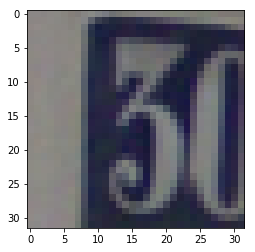Deep Learning Models -- A collection of various deep learning architectures, models, and tips for TensorFlow and PyTorch in Jupyter Notebooks.

In :
%load_ext watermark
%watermark -a 'Sebastian Raschka' -v -p torch

Sebastian Raschka

CPython 3.7.1
IPython 7.2.0

torch 1.0.0


# Model Zoo -- Using PyTorch Dataset Loading Utilities for Custom Datasets (Cropped Street View Hous Numbers, SVHN)¶

This notebook provides an example for how to load an image dataset, stored as individual PNG files, using PyTorch's data loading utilities. For a more in-depth discussion, please see the official

In this example, we are using the cropped version of the Street View House Numbers (SVHN) Dataset, which is available at http://ufldl.stanford.edu/housenumbers/.

To execute the following examples, you need to download the 2 ".mat" files

## Imports¶

In :
import pandas as pd
import numpy as np
import os

import torch
from torch.utils.data import Dataset
from torchvision import transforms

import matplotlib.pyplot as plt
from PIL import Image
import scipy.io as sio
import imageio


## Dataset¶

The following function will convert the images from ".mat" into individual ".png" files. In addition, we will create CSV contained the image paths and associated class labels.

In :
def make_pngs(main_dir, mat_file, label):

if not os.path.exists(main_dir):
os.mkdir(main_dir)

sub_dir = os.path.join(main_dir, label)
if not os.path.exists(sub_dir):
os.mkdir(sub_dir)

X = np.transpose(data['X'], (3, 0, 1, 2))
y = data['y'].flatten()

with open(os.path.join(main_dir, '%s_labels.csv' % label), 'w') as out_f:
for i, img in enumerate(X):
file_path = os.path.join(sub_dir, str(i) + '.png')
imageio.imwrite(os.path.join(file_path),
img)

out_f.write("%d.png,%d\n" % (i, y[i]))

make_pngs(main_dir='svhn_cropped',
mat_file='train_32x32.mat',
label='train')

make_pngs(main_dir='svhn_cropped',
mat_file='test_32x32.mat',
label='test')

In :
df = pd.read_csv('svhn_cropped/train_labels.csv', header=None, index_col=0)

Out:
1
0
0.png 1
1.png 9
2.png 2
3.png 3
4.png 2
In :
df = pd.read_csv('svhn_cropped/test_labels.csv', header=None, index_col=0)

Out:
1
0
0.png 5
1.png 2
2.png 1
3.png 10
4.png 6

## Implementing a Custom Dataset Class¶

Now, we implement a custom Dataset for reading the images. The __getitem__ method will

1. read a single image from disk based on an index (more on batching later)
2. perform a custom image transformation (if a transform argument is provided in the __init__ construtor)
3. return a single image and it's corresponding label
In :
class SVHNDataset(Dataset):

def __init__(self, csv_path, img_dir, transform=None):

self.img_dir = img_dir
self.csv_path = csv_path
self.img_names = df.index.values
self.y = df.values
self.transform = transform

def __getitem__(self, index):
img = Image.open(os.path.join(self.img_dir,
self.img_names[index]))

if self.transform is not None:
img = self.transform(img)

label = self.y[index]
return img, label

def __len__(self):
return self.y.shape


Now that we have created our custom Dataset class, let us add some custom transformations via the transforms utilities from torchvision, we

1. normalize the images (here: dividing by 255)
2. converting the image arrays into PyTorch tensors

Then, we initialize a Dataset instance for the training images using the 'quickdraw_png_set1_train.csv' label file (we omit the test set, but the same concepts apply).

Finally, we initialize a DataLoader that allows us to read from the dataset.

In :
# Note that transforms.ToTensor()
# already divides pixels by 255. internally

custom_transform = transforms.Compose([#transforms.Grayscale(),
#transforms.Lambda(lambda x: x/255.),
transforms.ToTensor()])

train_dataset = SVHNDataset(csv_path='svhn_cropped/train_labels.csv',
img_dir='svhn_cropped/train',
transform=custom_transform)

test_dataset = SVHNDataset(csv_path='svhn_cropped/test_labels.csv',
img_dir='svhn_cropped/test',
transform=custom_transform)

BATCH_SIZE=128

batch_size=BATCH_SIZE,
shuffle=True,
num_workers=4)

batch_size=BATCH_SIZE,
shuffle=False,
num_workers=4)


That's it, now we can iterate over an epoch using the train_loader as an iterator and use the features and labels from the training dataset for model training:

## Iterating Through the Custom Dataset¶

In :
device = torch.device("cuda:0" if torch.cuda.is_available() else "cpu")
torch.manual_seed(0)

num_epochs = 2
for epoch in range(num_epochs):

for batch_idx, (x, y) in enumerate(train_loader):

print('Epoch:', epoch+1, end='')
print(' | Batch index:', batch_idx, end='')
print(' | Batch size:', y.size())

x = x.to(device)
y = y.to(device)
break

Epoch: 1 | Batch index: 0 | Batch size: 128
Epoch: 2 | Batch index: 0 | Batch size: 128


Just to make sure that the batches are being loaded correctly, let's print out the dimensions of the last batch:

In :
x.shape

Out:
torch.Size([128, 3, 32, 32])

As we can see, each batch consists of 128 images, just as specified. However, one thing to keep in mind though is that PyTorch uses a different image layout (which is more efficient when working with CUDA); here, the image axes are "num_images x channels x height x width" (NCHW) instead of "num_images height x width x channels" (NHWC):

To visually check that the images that coming of the data loader are intact, let's swap the axes to NHWC and convert an image from a Torch Tensor to a NumPy array so that we can visualize the image via imshow:

In :
one_image = x.permute(1, 2, 0)
one_image.shape

Out:
torch.Size([32, 32, 3])
In :
# note that imshow also works fine with scaled
# images in [0, 1] range.
plt.imshow(one_image.to(torch.device('cpu')));In :
%watermark -iv

torch       1.0.0
pandas      0.23.4
imageio     2.4.1
numpy       1.15.4
torchvision 0.2.1
scipy       1.1.0
PIL.Image   5.3.0
matplotlib  3.0.2